## Winter News from MTSO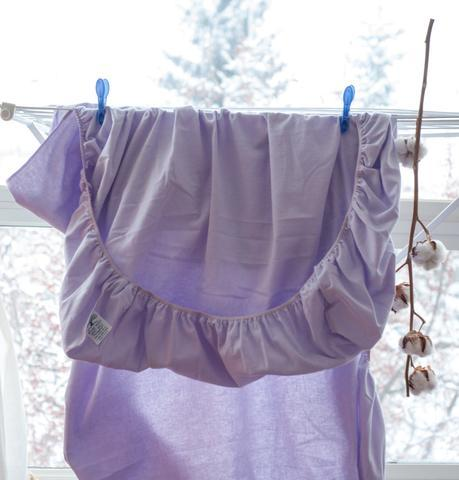Our popular flannelette sheets - perennial best sellers!

## Time to stock up and save.

Starting now we are offering great deals on oils and creams:
1. Biotone Advanced Therapy Gels, Lotions and Creams.
2. Bon Vital Original Oil - one Gallon - Buy two and get 20% off on the third.
3.Regular 2.8 L Coconut oil now on sale

 /* styles */ Looking for upgrades to your clinic or home office? Call Nutan at 780-440-1818 for suggestions on how to update your workspace with an affordable electric table, a sweet aromatherapy program, or new sheets and colourful winter blankets.
 table div table+table+table+table+table div table{width:100%;padding:0}table div table+table+table+table+table div table img{width:96.23%;padding:0;float:none}table div table+table+table+table+table div table td{width:100%;padding:0 1.88% 18px}/* styles */## DON'T MISS OUT - BIOTONE SALE!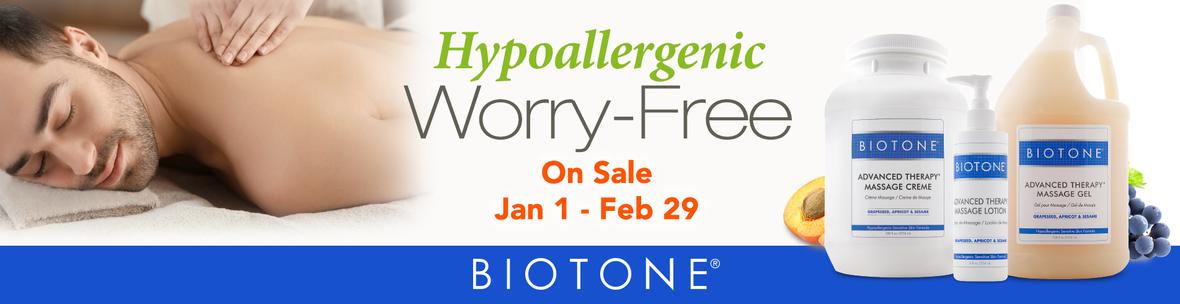ON SALE - BIOTONE ADVANCED THERAPY GELS, LOTIONS, CREAMS
 table div table+table+table+table+table+table+table+table+table div table{width:100%;padding:0}table div table+table+table+table+table+table+table+table+table div table img{width:96.23%;padding:0;float:none}table div table+table+table+table+table+table+table+table+table div table td{width:100%;padding:0 1.88% 18px}/* styles */## Bon Vital Original Massage Oil - SPECIAL OFFER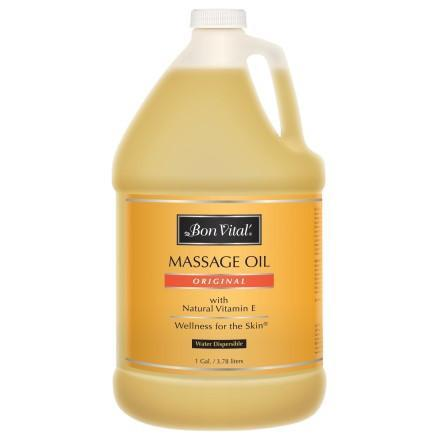Our thicker consistency allows for lasting glide, great for Stone massage, Lomilomi, or Swedish style modalities.

# Buy 2 gallons at regular price and get 20% off the third.

The blend of Grapeseed, Olive Oil, Jojoba, Avocado, Soybean, and Safflower oils make this the perfect mid-weight massage oil.

 table div table+table+table+table+table+table+table+table+table+table+table+table+table div table{width:100%;padding:0}table div table+table+table+table+table+table+table+table+table+table+table+table+table div table img{width:96.23%;padding:0;float:none}table div table+table+table+table+table+table+table+table+table+table+table+table+table div table td{width:100%;padding:0 1.88% 18px}/* styles */## The Beautiful Soft Scent of Coconut

 /* styles */ If you can't go to the tropics bring the tropics to your practice with MTSO Virgin Organic Coconut oil. Sale price until Feb.15th \$29.95
 table div table+table+table+table+table+table+table+table+table+table+table+table+table+table+table+table div table{width:100%;padding:0}table div table+table+table+table+table+table+table+table+table+table+table+table+table+table+table+table div table table{padding:0;float:left!important;width:29.056%!important}table div table+table+table+table+table+table+table+table+table+table+table+table+table+table+table+table div table table+table td,table div table+table+table+table+table+table+table+table+table+table+table+table+table+table+table+table div table td{padding-left:0;padding-right:0}table div table+table+table+table+table+table+table+table+table+table+table+table+table+table+table+table div table table td{padding-left:0;padding-right:20px}table div table+table+table+table+table+table+table+table+table+table+table+table+table+table+table+table div table table+table{float:left!important;width:70.944%!important}/* styles */
 table div table+table+table+table+table+table+table+table+table+table+table+table+table+table+table+table+table+table div table{width:100%;padding:0}table div table+table+table+table+table+table+table+table+table+table+table+table+table+table+table+table+table+table div table img{width:96.23%;padding:0;float:none}table div table+table+table+table+table+table+table+table+table+table+table+table+table+table+table+table+table+table div table td{width:100%;padding:0 1.88% 18px}/* styles */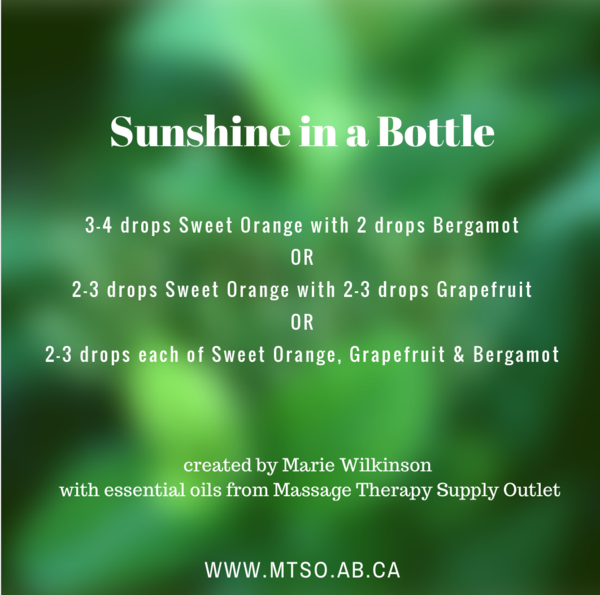Made with Essential Oils from MTSO - click the recipe for more info
 table div table+table+table+table+table+table+table+table+table+table+table+table+table+table+table+table+table+table+table+table div table{width:100%;padding:0}table div table+table+table+table+table+table+table+table+table+table+table+table+table+table+table+table+table+table+table+table div table img{width:96.23%;padding:0;float:none}table div table+table+table+table+table+table+table+table+table+table+table+table+table+table+table+table+table+table+table+table div table td{width:100%;padding:0 1.88% 18px}/* styles */# Amazing price on our featured lift-assist spa table

Pamper your clients with a super soft multi-adjustable, Stationary Spa Adjustable table. Feature price \$1199.00. This is the last one in stock! You'll love the beneficial effects of a professional spa table. Well-made, with back lift-assist that is easy to adjust and use. Perfect for your practice. (Featured sale table in black only, headrest and adjustable armrests sold separately.)

 table.module-21{width:72.26%;padding:0}table div table+table+table+table+table+table+table+table+table+table+table+table+table+table+table+table+table+table+table+table+table+table div table{width:72.26%;float:none;margin-left:auto;margin-right:auto;padding:0}table div table+table+table+table+table+table+table+table+table+table+table+table+table+table+table+table+table+table+table+table+table+table div table a{border:0 none;text-decoration:none}table div table+table+table+table+table+table+table+table+table+table+table+table+table+table+table+table+table+table+table+table+table+table div table img{width:100%!important;border:0 none;text-decoration:none}table div table+table+table+table+table+table+table+table+table+table+table+table+table+table+table+table+table+table+table+table+table+table div table td{width:100%;padding:0}/* styles */
 table div table+table+table+table+table+table+table+table+table+table+table+table+table+table+table+table+table+table+table+table+table+table+table+table div table{width:100%;padding:0}table div table+table+table+table+table+table+table+table+table+table+table+table+table+table+table+table+table+table+table+table+table+table+table+table div table img{width:96.23%;padding:0;float:none}table div table+table+table+table+table+table+table+table+table+table+table+table+table+table+table+table+table+table+table+table+table+table+table+table div table td{width:100%;padding:0 1.88% 18px}/* styles */# Towel Warmer Standard 120V UV

This dependable UL listed unit heats and sanitizes approximately 24 towels; it offers a sleek design featuring stainless steel interior, and a discrete drip pan. This unit offers a compact footprint design, that heats and sanitizes your cloth or disposable towels for continuous use throughout the day.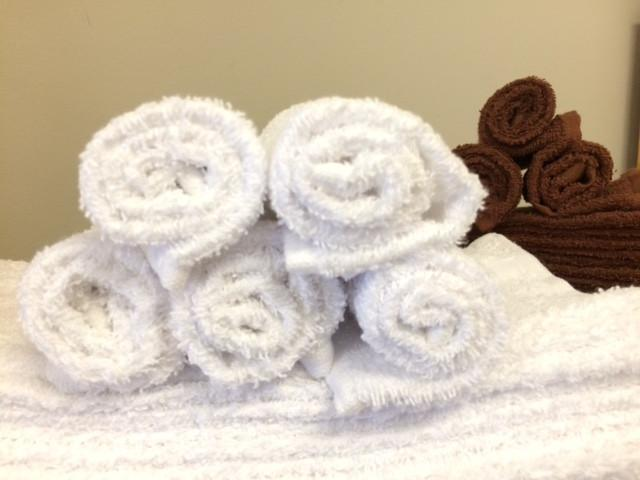Every practice needs a good towel warmer
 table div table+table+table+table+table+table+table+table+table+table+table+table+table+table+table+table+table+table+table+table+table+table+table+table+table+table+table+table div table{width:100%;padding:0}table div table+table+table+table+table+table+table+table+table+table+table+table+table+table+table+table+table+table+table+table+table+table+table+table+table+table+table+table div table img{width:96.23%;padding:0;float:none}table div table+table+table+table+table+table+table+table+table+table+table+table+table+table+table+table+table+table+table+table+table+table+table+table+table+table+table+table div table td{width:100%;padding:0 1.88% 18px}/* styles */Browse and buy from the extensive collection of quality products at our online store, always open, 24/7/365.

Drop by our Edmonton store and showroom: 10:00 - 6:00 Mon-Fri, 10:00-5:00 Sat.

 table.module-30{width:51.32%;padding:0}table div table+table+table+table+table+table+table+table+table+table+table+table+table+table+table+table+table+table+table+table+table+table+table+table+table+table+table+table+table+table+table div table{width:51.32%;float:none;margin-left:auto;margin-right:auto;padding:0}table div table+table+table+table+table+table+table+table+table+table+table+table+table+table+table+table+table+table+table+table+table+table+table+table+table+table+table+table+table+table+table div table a{border:0 none;text-decoration:none}table div table+table+table+table+table+table+table+table+table+table+table+table+table+table+table+table+table+table+table+table+table+table+table+table+table+table+table+table+table+table+table div table img{width:100%!important;border:0 none;text-decoration:none}table div table+table+table+table+table+table+table+table+table+table+table+table+table+table+table+table+table+table+table+table+table+table+table+table+table+table+table+table+table+table+table div table td{width:100%;padding:0}/* styles */
 Like   Tweet   Pin   in